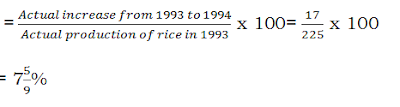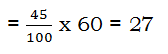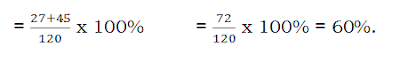New Students Offer - Use Code HELLO

# Quantitative Aptitude Percentage Tricks

### PERCENTAGES-

The word “Percent” indicates “for every hundred”. This idea is developed to facilitate easier evaluation of fractions by equalizing the denominator to hundred.

#### Example: -

7/11 as a percentage is represented asPercentages can also be represented as decimal fractions. In such a case, it is effectively equivalent to proportion of the original quantity.

Example
20% is the same as = 20/100 i.e., 0.2.
Any percentage can be stated as a decimal fraction by dividing the percentage figure by 100.

Ratio b/w any two quantities can also be expressed as a percentage.

#### Example

If the ratio of A & B is 3:2, we can say the ratio of A: B is 60%:40%.
Expressing x% as a fraction:-
X%= x out of 100=(x/100)
So, 75% = 75 out of 100 = (75/100) = (3/4)
Any percentage can be expressed as a decimal fraction by dividing the percentage figure by 100.

### Expressing decimal as a percentage:-

Any decimal fraction can be converted into percentage by multiplying it by 100.
0.5 = (0.5 x100)/100 = (50/100) =50%
0.25= (0.25 x100)/100 = (25/100) =25%
0.2= (0.2 x 100)/100= (20/100) = 20%

Note:-
1) When two numbers X & Y are given, then one number can be expressed as a percentage as a percentage of the other, in the following way.
X as a percent of Y = (X/Y) x 100
Y as a percent of X = (Y/X) x 100

2) X% of Y = Y% of X
Percentage Increase or Decrease:-
Percentage increase or decrease of a quantity is the ratio expressed in percentage of the actual increase or decrease of the quantity & the original amount of the quantity i.e.
Percentage IncreasePercentage decrease#### Example

If the production of rice went up from 225MT in 1993 to 242MT in 1994, then the percentage increase in rice production from1993 to 1994 is calculated as follows:
Actual Increase= 242-225=17 MT
Here this increase of 17MT is on 225 MT. (which is considered as the base for calculating the percentage increase).
Percentage IncreaseNote: - For calculating the percentage increase or decrease, the base is always the original quantity.
Whenever there is any percentage increase or decrease on a quantity, the new value of the quantity can be calculated directly, instead of actually calculating the increase / decrease on the original quantity & then adding to/ subtracting from the original quantity.

#### Example

If the increase on a figure of 350 is 15%, the new quantity is 1.15 x 350 = 402.5 (where 1.15 = 1+ 0.15, 0.15 being the decimal equivalent of 15%).
Similarly, if there is a decrease of 12% on a quantity of 225, then the new quantity will be equal to 225 x 0.88 = 198( where 0.88 = 1-0.12, 0.12 being the decimal equivalent of 12%).

### Examples:

1) The prices of rice at a shop in the months of July & August were ₹30 per kg & ₹ 36 per kg respectively. What is the percentage increase in the price of rice from July to August?
2) In an examination having 120 questions, Akhil answered 45% of the first 60 questions correctly & 75% of the next 60 questions correctly. What percentage of questions in the examination did he answer correctly?

Solution:- Number of questions Akhil answered correctly in first 60 questionsNumber of questions he answered correctly in the next 60 questionsPercentage of questions he answered correctly### Exercise Problems:-

1) Akhil donates 15% of his monthly income every month which is ₹ 1875. What is his annual income?
2) Raju spent ₹ 12024 to buy a washing machine, ₹ 8016 to buy a television & saved the rest amount of 25%. What was the total amount with raju?

Solutions:- 1) 3
2) 3